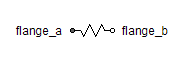Reset Integrator - MapleSim Help

Reset Integrator

Integrator block with resetDescription The Reset Integrator component computes, element-wise, the integral of the input $u$ with a multiplication gain of $k$. The integration is reset whenever input $r$ changes from $\mathrm{false}$ to $\mathrm{true}$. The Signal Size parameter assigns the dimension of the input and output connectors.
 Equations ${\stackrel{.}{y}}_{j}=k{u}_{j}$

Connections

 Name Description Modelica ID $u$ Real input vector u $y$ Real output vector y $r$ Boolean reset input r

Parameters

 Name Default Units Description Modelica ID k $1$ Integrator gain k Initial Values Initialization with initial states Type of initialization initType Signal Size $1$ Dimension of input and output signals signalSize ${y}_{0}$ $0$ Initial or guess value of output y_start

 Modelica Standard Library The component described in this topic is from the Modelica Standard Library. To view the original documentation, which includes author and copyright information, click here.